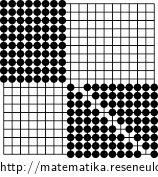## Goban stones II

There are several stones placed on the goban so that there are 8, 9 or 10 stones in each row and column.

How many stones can be left on the board so that at most one stone remains in each row and column?

The problem is difficult to calculate accurately, so it suffices to get lower and upper bounds that differ by 1.

• #### Hint

How many rows can be created that span fewer columns than the number of rows? Then generalize the reasoning and get an estimate of the deficit from the deficit Hall theorem.

• #### Solution

The lower bound can be obtained using the deficit Hall theorem: on $$r$$ lines there are at least $$8r$$ stones located in $$\lceil\frac8{10}r\rceil$$ columns. For $$r \le 19$$ we get $$\lceil\frac8{10}r\rceil=r-\lfloor\frac2{10}r\rfloor \ge r-3$$.

This is probably not the best lower bound. It is based on a situation where we would use only the first 16 columns in each of the 19 rows, but in fact we have to fill in the remaining three.

For an upper bound, a configuration can be found that allows the choice of only 17 stones: The first 10 columns are occupied by the first 8 columns; in the next 9 rows we will divide 10 stones into the last 11 columns so that each column has 8 or 9 stones, see picture.We can only leave 8 stones on the first 10 rows, which leads to a selection of a maximum of 17 stones.